# 【漆天编程】面向初学者的 MQL4 语言系列——MQL4 语言入门

### 首先要了解 MQL4

• 脚本就是命令序列，即您每请求一次才运行一次的程序。它们可替代您每天在交易中执行的操作。例如当您开订单时。它们也可执行特定功能，例如分析图表和生成统计信息。

• 定制指标是技术指标，主要作为内置指标的补充。它们用于开发图表或其他可视信息。和脚本不同的是，自定义指标在每次价格变动（即每跳动一次）时运行一次。显示什么指标只取决于您。它可以是一个没什么作用的窦道图，也可以是帮助您探寻市场方向的强大工具。例如，如果您确切地知道市场在什么时候、哪些情况下会趋于平盘态势，您可以将其编写到一个指标里。

• 是用于执行特定任务的函数集合。例如，您的某个 EA 可能使用了特殊的数学函数来决定买入和卖出的时机。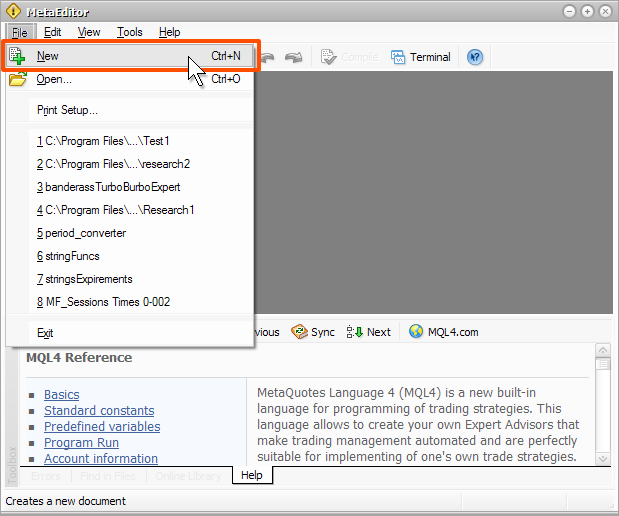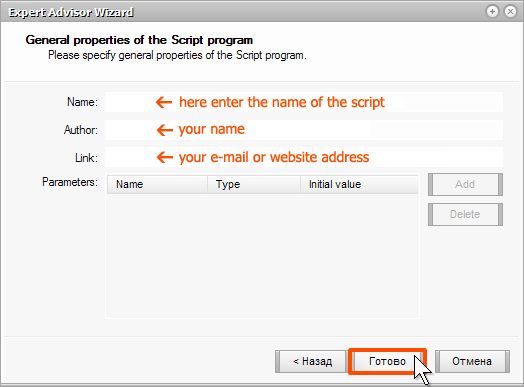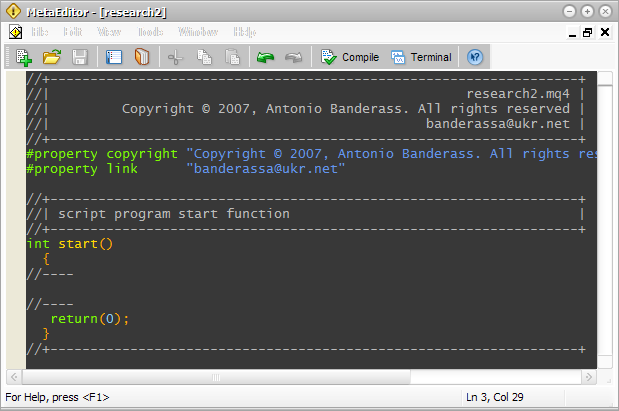### 源代码、编译和其他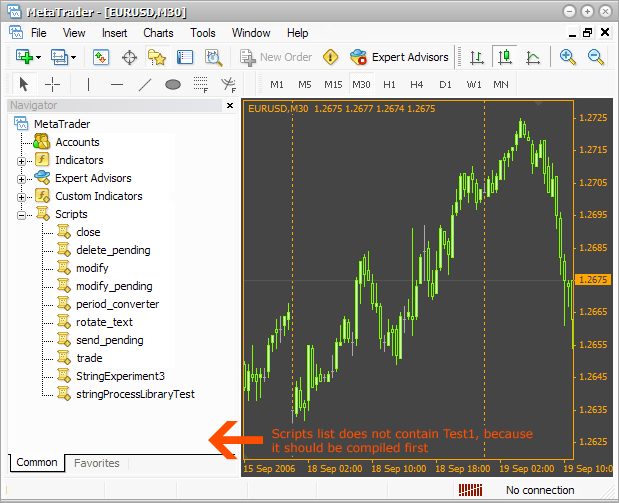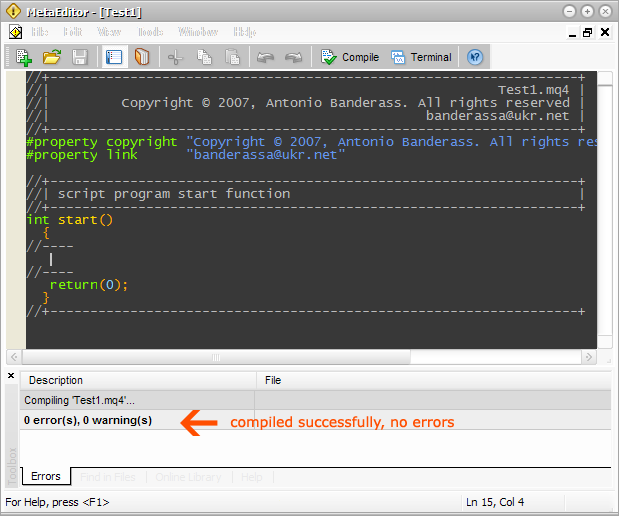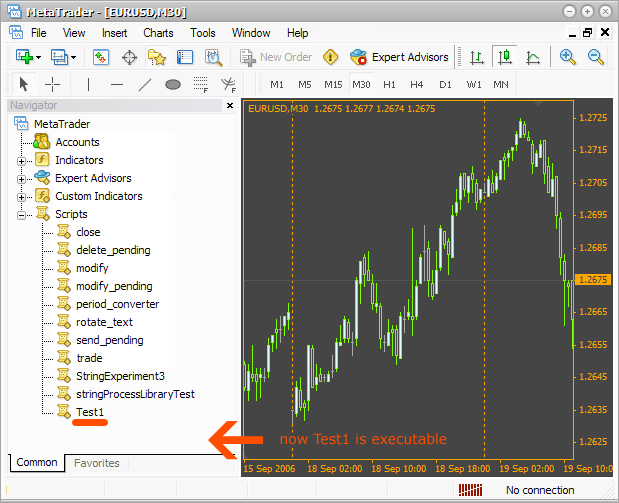### 应该在哪里编写脚本？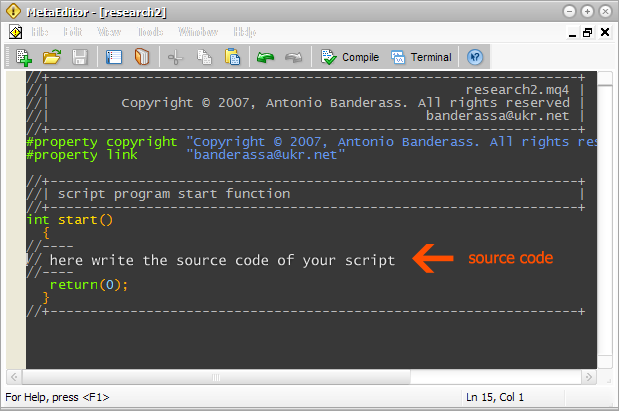### 变量

`int age = 25;`

[ 变量类型] [ 变量名称] = [ 变量]；

`int age;`

`double height = 1.95;`

`string name = "Janet";`

`bool trend = false;`

```double HIGHTPRICE;double hightprice;
double HightPrice;
double hightPrice;```

```DOUBLE hightPrice1;
Double hightPrice2;```

`double 1price;double %price;`

`// this is a comment`

### 使用变量

```double a = 50.0;// declare a value with a floating point and                // assign the value 50 to itdouble b = 2.0;
double c;

c = a + b;      // assign to the variable c sum of variables                // a and b. Now the value is equal to 52. Like after                // any other instruction put a semicolon (“;”) c = a - b;      // diminution, c = 48 c = a*b;        // multiplication, c = 100 c = a / b;      // division, c = 25   c = (a + b)*a;  // place the operations that should be performed                // first inside the brackets. In our case                 // first we get the sum of a and b                 // after that this sum will be multiplied by a and assigned to cc = (a + b) / (a - b); // if there are several operations in brackets,                 // they will be performed  c = a + b*3.0;  // according to mathematic rules first you will get                 // multiplication b and 3, and then the sum```

```int a = 5;
a = a + 5;  // add 5 a += 5;     // analogous a = a*5;
a *= 5;     // multiply a by 5 and assign to it a /= 5;     // divide and assign```

```int a = 5;
a++;  // add1, it is called incrementа--;  // subtract 1, it is decrement```

### MessageBox()

`MessageBox("Hello, World! There is some text.","caption");`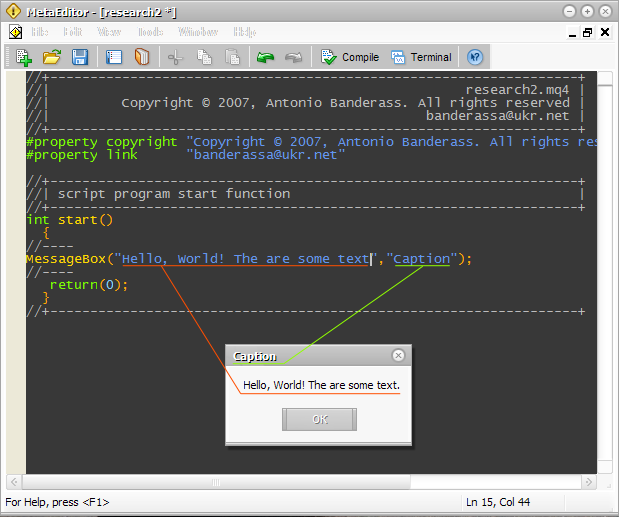```int a = 50;
int b = 100;
MessageBox("It is very simple. a+b=" + (a + b), "a+b=?")```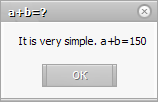```int a = 50;
int b = 100;
string str1 = "a + b =";
str1 += a + b; // now str1 = "a + b = 150"   // now use the variable str1 as // a first parameterMessageBox(str1, "a + b = ?");```

### 数组

```double price1 = 1.2341;
double price2 = 1.2321;
double price3 = 1.2361;
double price4 = 1.2411;
double price5 = 1.2301;```

`double price;`

（数组类型）（数组名称) [元素数量]；

```double price;   // declare an array of 5 elementsprice = 1.2341; // refer to the first element of the array and                    // assign a price to it. Note                    // that the index of the first element starts with 0                    // It is an important feature,                   // you should get used to it.   price = 1.2321;  // refer to the second element price = 1.2361;  // and so on price = 1.2411;
price = 1.2301;```

```double price;
price = 1.2234;
price = 1.2421;
MessageBox("Middle price is " + (price +
price) / 2.0,"middle price");```

`double price = {1.2234, 1.2421};`

`double price[] = {1.2234, 1.2421};`

### 集成或内置的数组和变量

`High;   // refer to the last maximal price,            // that the bar has achieved in the current timeframe            // and current currency pair. Currency pair and            // the timeframe depend on the chart, on which you            // have started a script. It is very important to remember! Low;    // minimal price of the last bar            // in the current chart. Volume; // value of the last bar in the current chart.`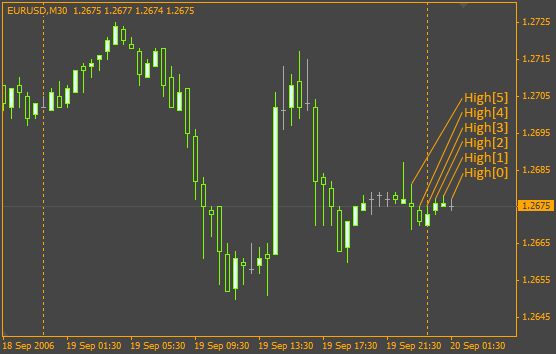### 循环

```double AveragePrice = 0.0;
AveragePrice += High;
AveragePrice += High;
AveragePrice += High;
AveragePrice += High;
AveragePrice += High;   // ... and so onAveragePrice /= Bars;```

```double AveragePrice = 0.0;
for(int a = 0; a < Bars; a++)
{
AveragePrice += High[a];
}```

`double AveragePrice = 0.0; // everything is clear// this is a cycle.or(int a = 0; a < Bars; a++)`

```for(declaration of a counter; the cycle operation conditions;
counter changes)
{
// the source code, that will be repeated is in braces,  }```

`a < Bars`

```// the cycle:// double AveragePrice=0.0;//// for(int a=0;a>// {//   AveragePrice+=High[a];// }//// will be performed in this way://double AveragePrice=0.0;int a=0;

AveragePrice+=High[a];
a++;                    // now a=1, suppose Bars=3.                        // then the cycle goes on, because Bars > a AveragePrice+=High[a];
a++;                   // a=2AveragePrice+=High[a];
а++;                   // a=3// the conditions is not fulfilled any more, so the cycle // stops, because a=3 and Bars=3```

`a>10    // the cycle works while a>10a!=10   // the cycle works while a is not equal to 10a==20   // while a is not equal to 20a>=2    // while a is more or equal to 2a<=30   // while a is less or equal to 30`

`a--     // the counter will each time decrease by 1 a += 2  // the counter will each time increase by 2`

```for(int a=0; a<Bars;)
{
AveragePrice+=High[a];

a++; // the counter changes inside the cycle body  }
>```

```int a = 0;
for(;a < Bars;)
{
AveragePrice += High[a];

a++; // the counter changes inside the cycle body
}```

```for(int a = 0; a < Bars; a++)
{
AveragePrice += High[a];
}```

```for(int a = 0; a < Bars; a++)
AveragePrice += High[a];```

### 条件

```// of course the conditionsn should be written in MQL4if( I have enough time )
{
// here we place any actions, directions in MQL4    will read this book;
}// if the first condition is not fulfilled, // the second is fulfilledelse
{
read a magazine;  // code  }```

```int a = 10;int b = 0;
if(a > 10 )
{
b = 1;
}else  {
b = 2;
}
MessageBox("b=" + b,".");```

```int a = 10;int b = 0;
if(a > 10)
{
b = 1;
}```

• int - 整数（1、60、772）；

• double - 浮点数（1.0021、0.221）；

• string - 仅行（“字”、“一些文本”）；

• bool - 仅 true 或 false（true、false）。

```int Integer=10;double Double=1.0;string String="JustWord";bool Bool=true;
if(Integer<10) // the condition is correct{
MessageBox("It works!","Really!");
}
if(Double!=1.0) // the condition is correct{
MessageBox("works!","Simple!");
}
if(String==10) // this is nonsense!!                // we found the variable                //String with string type, but 10 is int{
MessageBox("Wrong type","u can't c this");
}
if(String!="Word") // ok{{
// ...}
if(Bool==true) // correct{
// ...}```

```int a=0;double b=0.0;bool e;
if(a==0)
{
for(int c=0;c<Bars;c++)
{
b+=High[c];
}

if(b>500.0)
{
e=true;
}
else  {
e=false;
}
}```

```int a=0;int result;
if(a==0)
{
result=1;
}else if(a==1)
{
result=2;
}else if(a==2)
{
result=3;
}else{
result=4;
}```

```if(a==1)
{
b=2;
}

// or this way:if(a==1)
b=2;```

### 复杂条件

```if((enough time) && (enough oatience))
{
I will learn MQL4 language;
}```

```nt a=10;int b=20;
if((a>5) && (b<50))
MessageBox("Its works!","Yes");```

```int a=10;int b=20;
if ( ((a>5) || (a<15)) && ( b==20))
MessageBox("It works, too!","Yes");```

### 其他内置变量和数组

```double Open[]  // the array of prices of the current               // chart opening bars
double Close[] // the array of prices of the current                // chart closing bars
double Bid  // the last known buying price in             // the current currency pair
double Ask  // the last known selling price in            // the current currency pair```

### 总结

#### 评论